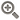﻿ 什么是线性回归斜率公式？-点掌财经• 综合
• 股票
• 板块
• 嘉宾
• 课程
• 基金
• 经理
• 说说
• 掌评
• 消息
• 好看
• 话题

# 什么是线性回归斜率公式？经典RL:=(CLOSE-LLV(LOW,9))/(HHV(HIGH,9)-LLV(LOW,9))*100;

经典K:=SMA(经典RL,3,1); 经典D:=SMA(经典K,3,1); 经典J:=3*经典K-2*经典D;

MAHL1:=100*((EMA((H+L)/2,3)-LLV(EMA((H+L)/2,5),30)-(EMA(H,20)-EMA(L,20))) /(LLV(EMA((H+L)/2,5),30)-(EMA(H,20)-EMA(L,20))));

MAHL2:=IF(EMA(C,2)>回归斜率线A AND MAHL1>0,EMA(C,3),LLV(EMA((H+L)/2,5),30));{A}

MAHL3:=IF(EMA(C,2)>回归斜率线A AND MAHL1>0,LLV(EMA((H+L)/2,5),30),EMA(C,5));{A}

{回归斜率带:DRAWBAND(回归斜率线A,RGB(235,0,230),REF(回归斜率线A,2),RGB(0,104,154));}

上线:=SMA(C,6.5,1);

一号支撑线:SMA(C,14.5,1);

上线1:=SMA(C,3,1);

一号支撑线1:=SMA(C,8,1);

上线2:=SMA(C,3.5,1);

DRAWTEXT(CROSS(一号支撑线1,上线1),H*1.08,’洗\n盘’),COLOR00FF00;

BBI:=(MA(CLOSE,3)+MA(CLOSE,6)+MA(CLOSE,12)+MA(CLOSE,25))/4;

UPR:=BBI+3*STD(BBI,13)。

这只是其中一部分的公式，小编之所以没有将全部的公式放出来，主要是因为不同的操作要求，公式的内容也会有所区别。所以对于公式大家可以参考，但是不能照搬。大家在编写公式的时候，需要根据股票k线的实时走势来编写出公式，这样才能准确的知道自己的买入点卖出点在哪里。

#### 最新发布• 手机号码：

请输入手机号码，您的个人信息严格保密，请放心

• 图形验证码：

请输入右侧图形验证码

• 短信验证码：

请输入接收的短信验证码

获取短信验证码
X• 10牛
• 30牛
• 50牛
• 其它